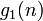# Dixon's theorem

## Statement

Let$S_n$ denote the symmetric group of degree$n$, and$A_n$ denote the subgroup that is the alternating group. Pick two elements independently of each other, both uniformly at random, from$S_n$. Denote by$f_1(n)$ the probability that they generate all of$S_n$, and by$f_2(n)$ the probability that they generate$A_n$.

Then:$\lim_{n \to \infty} f_1(n) = \frac{3}{4}$

and:$\lim_{n \to \infty} f_2(n) = \frac{1}{4}$

Thus:$\lim_{n \to \infty} f_1(n) + f_2(n) = 1$

Another way of putting this is as follows:

• Define by$g_1(n)$ the probability that two permutations generate the whole group conditional to the elements not both being even permutations. Then, clearly$f_1(n) = (3/4)g_1(n)$. The first part of Dixon's theorem states that$\lim_{n \to \infty} g_1(n) = 1$.
• Define by$g_2(n)$ the probability that two permutations generate the whole group conditional to the elements both being even permutations. Then, clearly$f_2(n) = (1/4)g_2(n)$. The second part of Dixon's theorem states that$\lim_{n \to \infty} g_2(n) = 1$.